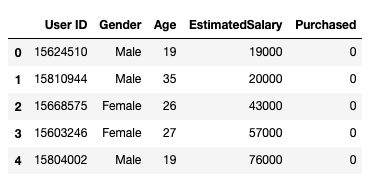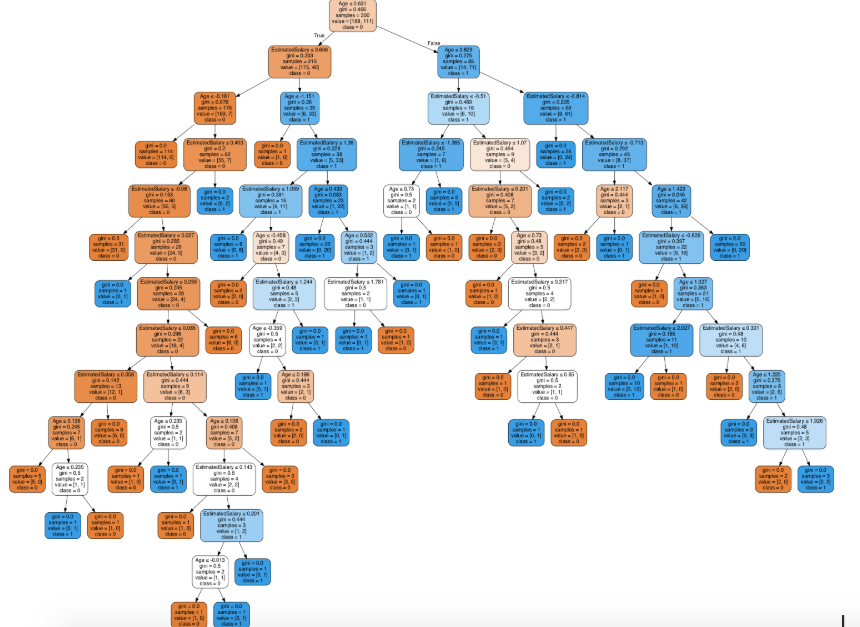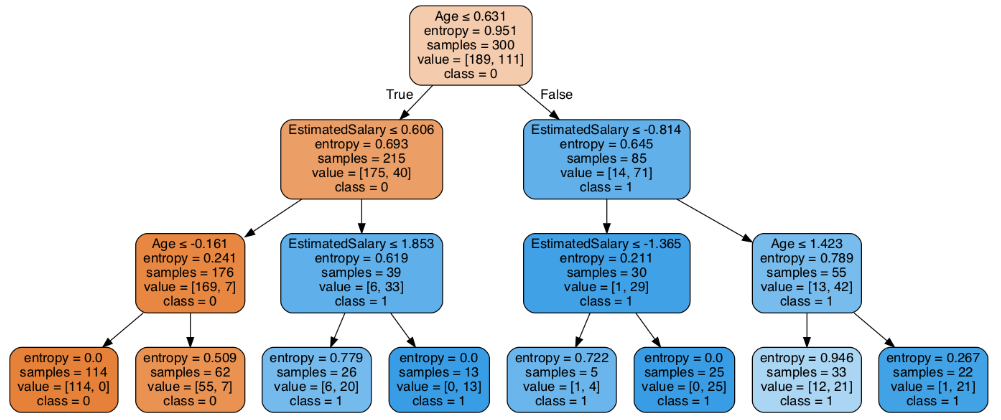The decision of making strategic splits heavily affects a tree’s accuracy. Regression trees and classification trees use different criteria for making decisions.

In decision trees, multiple algorithms are used to determine whether a node should be split into two or more sub-nodes. The creation of sub-nodes increases their homogeneity. We can therefore say that the purity of the node increases as the target variable increases.

A decision tree divides the nodes according to all the available variables and selects the split that produces the most homogeneous sub-nodes.

A selection of algorithms is also based on the type of variables to be targeted. Here are some algorithms used in Decision Trees:

ID3 → (extension of D3)

C4.5 → (successor of ID3)

CART → (Classification And Regression Tree)

CHAID → (Chi-square automatic interaction detection Performs multi-level splits when computing classification trees)

MARS → (multivariate adaptive regression splines)

Based on a top-down greedy search through the space of possible branches without turning back, the ID3 algorithm builds decision trees. The greedy algorithm, as its name suggests, makes the best decision at the time.

### Steps in ID3 algorithm:

1. As a starting point, the original set S is used.

2. Every iteration of the algorithm calculates the Entropy(H) and Information gain(IG) of the very unused attributes of the set S.

3. In the next step, the attribute with the smallest entropy or largest information gain is selected.

4. The set S is then split by the selected attribute to produce a subset of the data.

5. With each subset, the algorithm recurs, considering only attributes that have never been considered before.

## Attribute Selection Measures

Choosing which attribute to place at the root or at different levels of the tree as internal nodes can be difficult if the dataset has N attributes. It is not possible to solve the problem by randomly selecting any node as the root. We may get bad results with low accuracy if we follow a random approach.

The problem of attribute selection was solved by researchers who devised some solutions. They suggested using criteria such as:

Entropy,

Information gain,

Gini index,

Gain Ratio,

Reduction in Variance

Chi-Square

Each attribute will be valued based on these criteria. According to the sort order, attributes are placed in the tree based on their values, i.e., the attribute with the highest value (in the case of information gain) is placed at the top.

We assume categorical attributes for Information Gain, and continuous attributes for Gini index.

### Entropy

Entropy measures how random information is being processed. An increase in entropy makes it harder to draw any conclusions from the information. For example. Random information can be obtained by flipping a coin.

Based on the above graph, it is quite evident that the entropy H(X) is zero when the probability is either 0 or 1. If the probability is 0.5, the Entropy of the data is maximum since perfect randomness is projected and there is no chance of predicting the outcome perfectly.

According to ID3, a branch with an entropy of zero is a leaf node, while a branch with an entropy greater than zero requires further splitting.

Entropy is mathematically represented as:

Where S → Current state, and Pi → Probability of an event of state S or Percentage of class i in a node of state S.

Mathematically Entropy for multiple attributes is represented as:

where T→ Current state and X → Selected attribute

### Information Gain

A statistical property called information gain or IG measures how well a given attribute separates training examples according to their target classification. The key to constructing a decision tree is to select the attribute with the greatest information gain and smallest entropy.

Information Gain

An increase in information leads to a decrease in entropy. Based on given attribute values, it computes the difference between entropy before split and average entropy after split. Information gain is used in the ID3 (Iterative Dichotomiser) decision tree algorithm.

IG can be represented mathematically as follows:

We can conclude, much more simply, that:

In this example, “before” represents the dataset before splitting, K represents the number of subsets produced by splitting, and (j, after) represents subset j after splitting.

### Gini Index

Gini indexes are cost functions used to evaluate splits in datasets. To calculate it, subtract the squared probabilities of each class from one. It favors large partitions that are easier to implement, whereas information gain favors smaller partitions with distinct values.

Gini Index

The Gini Index uses the categorical variable “Success” or “Failure”. It only performs binary splits.

Gini index values above 1.0 indicate greater inequality and heterogeneity.

### Steps to Calculate Gini index for a split

1. Calculate Gini for sub-nodes, using the above formula for success(p) and failure(q) (p²+q²).

2. Calculate the Gini index for split using the weighted Gini score of each node of that split.

CART (Classification and Regression Tree) uses the Gini index method to create split points.

### Gain ratio

There is a bias in information gain towards choosing attributes with a large number of values as root nodes. Essentially, it prefers attributes with a large number of distinct values.

Gain ratio is a modification of Information gain, which reduces its bias and is usually the best option in C4.5, an improvement of ID3. By taking into account the number of branches that would result before dividing, gain ratio overcomes the problem with information gain. An intrinsic information of a split is taken into account when correcting information gain.

What if we had a dataset with users and their movie genre preferences based on variables like gender, age group, rating, etc. Based on information gain, you split at ‘Gender’ (assuming it has the greatest information gain) and now ‘Group of Age’ and ‘Rating’ may have equal significance. Through the use of gain ratio, a variable with more distinct values will be penalized, which will help us determine where to split.

Gain Ratio

The dataset before the split is called “before”, K is the number of subsets generated by the split, and (j, after) is the subset j after the split.

### Reduction in Variance

A reduction in variance algorithm is used for continuous target variables (regression problems). To determine the best split, the standard variance formula is used. Using the split with the lowest variance as a criterion for splitting the population, we select the split with the lowest variance:

As shown above, X-bar is the mean of the values, X is the actual value, and n is the number of values.

Steps to calculate Variance:

1. For each node, calculate the variance.

2. Calculate the variance for each split as the weighted average of each node’s variance.

### Chi-Square

The acronym CHAID stands for Chi-squared Automatic Interaction Detector. It is one of the oldest methods for classifying trees. Sub-nodes and their parent nodes are compared statistically to determine whether there is a significant difference between them. The sum of squared differences between observed and expected frequencies of the target variable is used to measure it.

“Success” or “Failure” is the categorical target variable. Splits can be performed in two or more directions. The higher the value of Chi-Square, the greater the statistical significance of differences between the sub-node and parent node.

It creates a tree called CHAID (Chi-square Automatic Interaction Detector).

Mathematically, Chi-squared is represented as:

### Steps to Calculate Chi-square for a split:

1. The deviation of Success and Failure for an individual node is used to calculate Chi-square

2. Using the sum of successful and unsuccessful Chi-squares at each node of the split, calculate the Chi-square of the split

## How to avoid/counter Overfitting in Decision Trees?

It is common for Decision Trees to fit a lot into a table, especially when it has a lot of columns. Some of the training data set seems to have been memorized by the tree. In the worst case scenario, a decision tree will make one leaf for each observation, so it will give you 100% accuracy on your training data set. The accuracy of predictions for samples that aren’t in the training set is affected by this.

Here are two ways to remove overfitting:

1. Pruning Decision Trees.
2. Random Forest

### Pruning Decision Trees

Trees grow until they reach the stopping criteria as a result of the splitting process. However, the fully grown tree is likely to overfit the data, resulting in poor accuracy.

Pruning is the process of trimming off the branches of the tree, i.e., removing the decision nodes from the leaf nodes so as not to disturb the overall accuracy of the tree. Using the segregated training data set, D, prepare the decision tree. Then segment the training data set into two parts: training data set, D, and validation data set, V. Continue trimming the tree accordingly to optimize the accuracy of the validation data set.

Pruning

Due to its greater importance on the right-hand side of the tree, the ‘Age’ attribute on the left hand side of the tree has been pruned, preventing overfitting.

## Random Forest

To achieve better predictive performance, Random Forest combines multiple machine learning algorithms.

### Why the name “Random”?

Two key concepts that give it the name random:

1. When building trees, a random sampling of training data is taken.

2. When splitting nodes, random subsets of features are taken into account.

Using a technique called bagging, multiple training sets are generated with replacements to create an ensemble of trees.

The bagging technique uses randomized sampling to divide a data set into N samples. Then, using a single learning algorithm a model is built on all samples. Later, the resultant predictions are combined using voting or averaging in parallel.

## Which is better Linear or tree-based models?

Well, it depends on the kind of problem you are solving.

1. Linear regression outperforms tree-based models if they can well approximate the relationships between dependent and independent variables.

2. Whenever there is a high degree of non-linearity and complex relationship between dependent and independent variables, a tree model will perform better than a classical regression model.

3. A decision tree model is always better to explain to people than a linear model if you want an easy-to-understand model. Decision tree models are even simpler to interpret than linear regression!

### Decision Tree Classifier Building in Scikit-learn

The dataset that we have is a supermarket data which can be downloaded from here.

```import numpy as np
import matplotlib.pyplot as plt
import pandas as pd```

Load the dataset. It consists of 5 features, `UserID``Gender``Age``EstimatedSalary` and `Purchased`.

```data = pd.read_csv('/Users/ML/DecisionTree/Social.csv')### Dataset

We will take only `Age` and `EstimatedSalary` as our independent variables `X` because of other features like `Gender` and `User ID` are irrelevant and have no effect on the purchasing capacity of a person. Purchased is our dependent variable `y`.

```feature_cols = ['Age','EstimatedSalary' ]X = data.iloc[:,[2,3]].values
y = data.iloc[:,4].values```

The next step is to split the dataset into training and test.

```from sklearn.model_selection import train_test_split
X_train, X_test, y_train, y_test =  train_test_split(X,y,test_size = 0.25, random_state= 0)```

Perform feature scaling

```#feature scaling
from sklearn.preprocessing import StandardScaler
sc_X = StandardScaler()
X_train = sc_X.fit_transform(X_train)
X_test = sc_X.transform(X_test)```

Fit the model in the Decision Tree classifier.

```from sklearn.tree import DecisionTreeClassifier
classifier = DecisionTreeClassifier()
classifier = classifier.fit(X_train,y_train)```

Make predictions and check accuracy.

```#prediction
y_pred = classifier.predict(X_test)#Accuracy
from sklearn import metricsprint('Accuracy Score:', metrics.accuracy_score(y_test,y_pred))```

The decision tree classifier gave an accuracy of 91%.

Confusion Matrix

```from sklearn.metrics import confusion_matrix
cm = confusion_matrix(y_test, y_pred)Output:
array([[64,  4],
[ 2, 30]])```

It means 6 observations have been classified as false.

Let us first visualize the model prediction results.

```from matplotlib.colors import ListedColormap
X_set, y_set = X_test, y_test
X1, X2 = np.meshgrid(np.arange(start = X_set[:,0].min()-1, stop= X_set[:,0].max()+1, step = 0.01),np.arange(start = X_set[:,1].min()-1, stop= X_set[:,1].max()+1, step = 0.01))
plt.contourf(X1,X2, classifier.predict(np.array([X1.ravel(), X2.ravel()]).T).reshape(X1.shape), alpha=0.75, cmap = ListedColormap(("red","green")))plt.xlim(X1.min(), X1.max())
plt.ylim(X2.min(), X2.max())for i,j in enumerate(np.unique(y_set)):
plt.scatter(X_set[y_set==j,0],X_set[y_set==j,1], c = ListedColormap(("red","green"))(i),label = j)
plt.title("Decision Tree(Test set)")
plt.xlabel("Age")
plt.ylabel("Estimated Salary")
plt.legend()
plt.show()```

Let us also visualize the tree:

You can use Scikit-learn’s export_graphviz function to display the tree within a Jupyter notebook. For plotting trees, you also need to install Graphviz and pydotplus.

`conda install python-graphviz`
`pip install pydotplus`

export_graphviz function converts decision tree classifier into dot file and pydotplus convert this dot file to png or displayable form on Jupyter.

```from sklearn.tree import export_graphviz
from sklearn.externals.six import StringIO
from IPython.display import Image
import pydotplusdot_data = StringIO()
export_graphviz(classifier, out_file=dot_data,
filled=True, rounded=True,
special_characters=True,feature_names = feature_cols,class_names=['0','1'])
graph = pydotplus.graph_from_dot_data(dot_data.getvalue())
Image(graph.create_png())```Decision Tree.

In the decision tree chart, each internal node has a decision rule that splits the data. Gini referred to as the Gini ratio, which measures the impurity of the node. You can say a node is pure when all of its records belong to the same class, such nodes known as the leaf node.

Here, the resultant tree is unpruned. This unpruned tree is unexplainable and not easy to understand. In the next section, let’s optimize it by pruning.

Optimizing the Decision Tree Classifier

criterion: optional (default=”gini”) or Choose attribute selection measure: This parameter allows us to use the different-different attribute selection measure. Supported criteria are “gini” for the Gini index and “entropy” for the information gain.

splitter: string, optional (default=”best”) or Split Strategy: This parameter allows us to choose the split strategy. Supported strategies are “best” to choose the best split and “random” to choose the best random split.

max_depth: int or None, optional (default=None) or Maximum Depth of a Tree: The maximum depth of the tree. If None, then nodes are expanded until all the leaves contain less than min_samples_split samples. The higher value of maximum depth causes overfitting, and a lower value causes underfitting (Source).

In Scikit-learn, optimization of decision tree classifier performed by only pre-pruning. The maximum depth of the tree can be used as a control variable for pre-pruning.

```# Create Decision Tree classifer object
classifier = DecisionTreeClassifier(criterion="entropy", max_depth=3)# Train Decision Tree Classifer
classifier = classifier.fit(X_train,y_train)#Predict the response for test dataset
y_pred = classifier.predict(X_test)# Model Accuracy, how often is the classifier correct?
print("Accuracy:",metrics.accuracy_score(y_test, y_pred))```

Well, the classification rate increased to 94%, which is better accuracy than the previous model.

Now let us again visualize the pruned Decision tree after optimization.

```dot_data = StringIO()
export_graphviz(classifier, out_file=dot_data,
filled=True, rounded=True,
special_characters=True, feature_names = feature_cols,class_names=['0','1'])
graph = pydotplus.graph_from_dot_data(dot_data.getvalue())
Image(graph.create_png())```Decision Tree after pruning

This pruned model is less complex, explainable, and easy to understand than the previous decision tree model plot.

## Conclusion

In this article, we have covered a lot of details about Decision Tree; It’s working, attribute selection measures such as Information Gain, Gain Ratio, and Gini Index, decision tree model building, visualization and evaluation on supermarket dataset using Python Scikit-learn package and optimizing Decision Tree performance using parameter tuning.